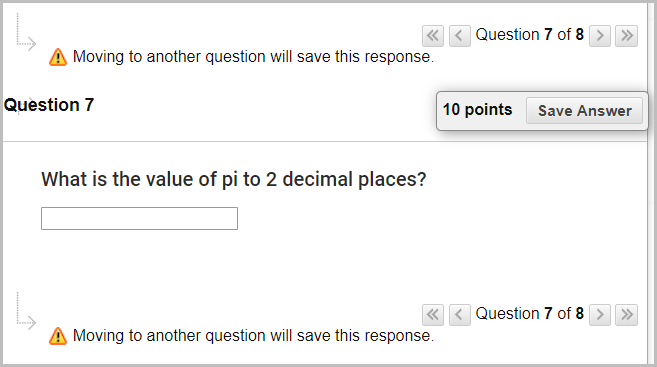# Learn.UQ Quizzes, Tests and Exams Online Workshop

## 11. Create Calculated Numeric Question

### Overview

Calculated Numeric Questions are useful in that students only need to enter numerals for their answer and does not require the use of a mathematical formula. In addition to that, you can provide a text question that requires a numeric answer.

### Example question

What is the value of pi to 2 decimal places?### Create a Calculated Numeric Question

1. In your Learn.UQ course, from the Control Panel, select Course Tools > Tests, Surveys and Pools > Pools.
2. Click on the drop-down arrow next to Test Pool [your full name] then select Edit.
3. In your Pool, click on Create Question > Calculated Numeric.
4. Enter "What is the value of pi to 2 decimal places?" in the Question Text textbox.
5. Enter "3.14" in the Correct Answer textbox.
6. Click on the Submit button.